Question

At a carnival, you can try to ring a bell by striking a target with a 11.7-kg hammer. In response, a 0.378-kg metal piece is sent upward toward the bell, which is 5.13 m above. Suppose that 18.0 percent of the hammer’s kinetic energy is used to do the work of sending the metal piece upward. How fast must the hammer be moving when it strikes the target so that the bell just barely rings

1.Thunguyet

Explanation:

mass of hammer, Mh = 11.7 kg

mass of metal, Mm = 0.378 kg

height, h = 5.13 m

According to the transformation of energy

Potential energy of metal piece = 18% of kinetic energy of hammer

Mm x g x h = 18 % of 0.5 x Mh x v²

where, v is the velocity of hammer

0.378 x 9.8 x 5.3 = 0.18 x 0.5 x 11.7 x v²

v² = 18.65

v = 4.31 m/s

Thus, the velocity of hammer is 4.31 m/s.

2.4.25035202162 m/s

Explanation: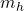= Mass of hammer = 11.7 kg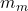= Mass of metal piece = 0.378 kg

g = Acceleration due to gravity =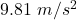h = Height = 5.13 m

Energy required to raise the bell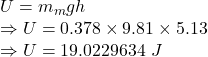From the question we have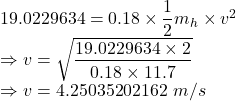The speed of the metal piece is 4.25035202162 m/s